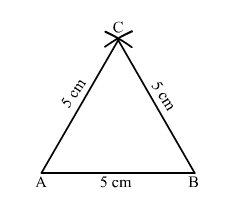# Construct an equilateral triangle each of whose sides measures 5 cm.

Question:

Construct an equilateral triangle each of  whose sides measures 5 cm.

Solution:Steps of construction:
1. Draw a line segment AB = 5 cm.
2. With A as the centre and a radius equal to AB, draw an arc.
3. With B as the centre and the same radius as above, draw another arc cutting the previously drawn arc at C.
4. Join AC and BC.

Thus, $\triangle A B C$ is the required triangle.